# 2.9 – Example

Previous: 2.8 – Expected Value, Variance, Standard Deviation

Next: 2.10 – Lesson 2 Summary

## Problem

The length of time X, needed by students in a particular course to complete a 1 hour exam is a random variable with PDF given by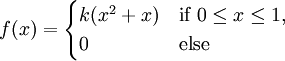For the random variable X,

1. Find the value k that makes f(x) a probability density function (PDF)
2. Find the cumulative distribution function (CDF)
3. Graph the PDF and the CDF
4. Use the CDF to find
1. Pr(X ≤ 0)
2. Pr(X ≤ 1)
3. Pr(X ≤ 2)
5. find the probability that that a randomly selected student will finish the exam in less than half an hour
6. Find the mean time needed to complete a 1 hour exam
7. Find the variance and standard deviation of X

## Solution

### Part 1

The given PDF must integrate to 1. Thus, we calculate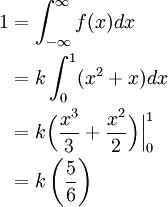Therefore, k = 6/5.

### Part 2

The CDF, F(x), is area function of the PDF, obtained by integrating the PDF from negative infinity to an arbitrary value x.

If x is in the interval (-∞, 0), thenIf x is in the interval [0, 1], then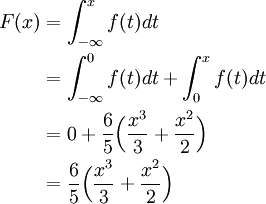If x is in the interval (1, ∞) then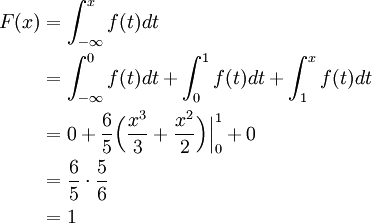Note that the PDF f is equal to zero for x > 1. The CDF is therefore given by### Part 3

The PDF and CDF of X are shown below.

### Part 4

These probabilities can be calculated using the CDF: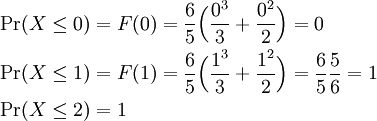Note that we could have evaluated these probabilities by using the PDF only, integrating the PDF over the desired event.

### Part 5

The probability that a student will complete the exam in less than half an hour is Pr(X < 0.5). Note that since Pr(X = 0.5) = 0, since X is a continuous random variable, we an equivalently calculate Pr(x ≤ 0.5). This is now precisely F(0.5):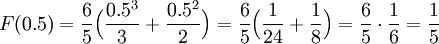### Part 6

The mean time to complete a 1 hour exam is the expected value of the random variable X. Consequently, we calculate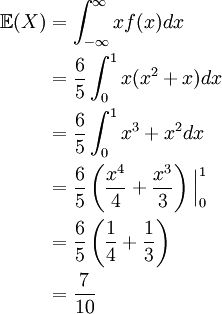### Part 7

To find the variance of X, we use our alternate formula to calculate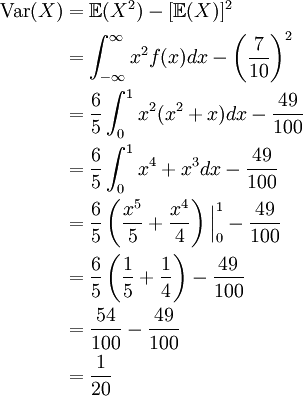Finally, we see that the standard deviation of X is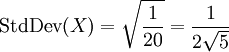Previous: 2.8 – Expected Value, Variance, Standard Deviation

Next: 2.10 – Lesson 2 Summary